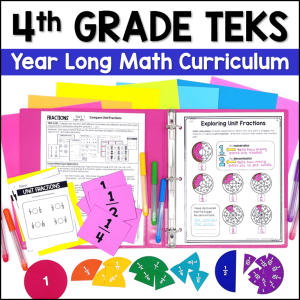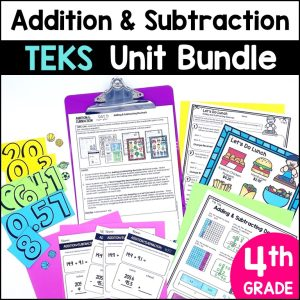Save 10% on your first order with code EXTRA10

# Estimating Sums and Differences – Rounding Google Slides – 3 to 6-digit Numbers

\$2.50187.25\$22.00
SKU: 6063120 Category:

## Description

This resource includes 10 interactive Google Slides and video instructions for students. In this activity, students will practice rounding to the nearest hundred, thousand, ten thousand or hundred thousand to estimate sums and differences.

This resource can be easily assigned in Google Classroom for math stations, independent practice or distance learning. You do not need Google Classroom to use this resource, but you do need a Google e-mail to use Google Slides.

What’s Included?

• Video Instructions (Student devices must be able to access You-Tube)
• 20 problems where students estimate to find sums and differences of 3 to 6-digit numbers
• Key

Topics include:

• Rounding to the nearest hundred, thousand, ten thousand and hundred thousand
• Adding and subtracting two numbers (up to 6-digit numbers)
• Repetitive practice using the terms “sum” and “difference” to help students memorize the definitions of those terms

Ideas on how to use this resource:

• Use it as a whole class teaching tool by projecting the slides and using the movable, interactive pieces to teach your lesson
• Use this resource as a no prep math station
• Use this resource for small group reteach or tutoring
• Assign this resource as a lesson for student practice as independent work
• Share the digital key for parents and students to check work at home